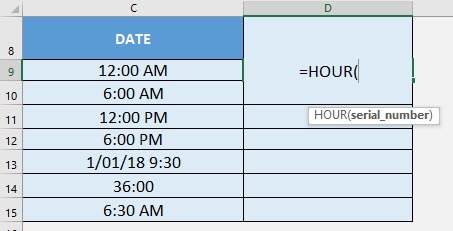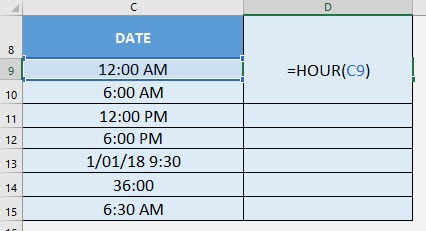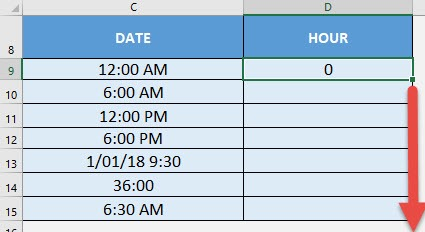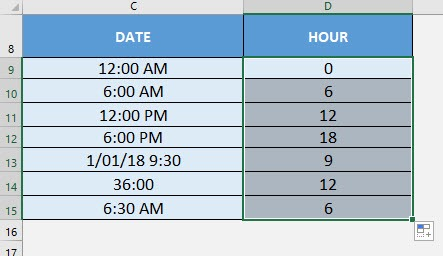What does it do?

Gets the hour from the time

Formula breakdown:

=HOUR(serial_number)

What it means:

=HOUR(time wherein the hour will be extracted from)

I recall wherein I had a lot of times and I wanted to extract the hour of the times one-by-one… It was too much of a hassle! Thankfully there is Excel’s HOUR Formula to do that for me!

A couple of interesting things on the HOUR Formula:

• The hour it returns to you is similar to military time ranging from 0 – 23
• If it’s a date time, then the date gets ignored
• If the time is greater than 24 hours, then it simply converts it to days and hours, then returns the hour component only

I explain how you can do this below:STEP 1: We need to enter the HOUR function in a blank cell:

## =HOUR(STEP 2: The HOUR arguments:

## serial_number

What is the time that you want to extract the hour from?

Select the cell containing the time:

## =HOUR(C9)Apply the same formula to the rest of the cells by dragging the lower right corner downwards.You now have your years extracted!How to Use the HOUR Formula in Excel